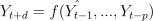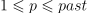# module timeseries.base#

## Short summary#

module mlinsights.timeseries.base

Base class for timeseries.

source on GitHub

## Classes#

class

truncated documentation

BaseReciprocalTimeSeriesTransformer

Base for all timeseries preprocessing automatically applied within a predictor.

BaseTimeSeries

Base class to build a predictor on timeseries. The class computes one or several predictions at each time, between …

TimeSeriesRegressorMixin

## Properties#

property

truncated documentation

_repr_html_

HTML representation of estimator. This is redundant with the logic of _repr_mimebundle_. The latter should …

_repr_html_

HTML representation of estimator. This is redundant with the logic of _repr_mimebundle_. The latter should …

## Methods#

method

truncated documentation

__init__

__init__

_applies_preprocessing

Applies the preprocessing to the series.

_applies_preprocessing_inv

Applies the preprocessing to the series.

_base_fit_predict

Trains the preprocessing and returns the modified X, y, sample_weight.

_fit_preprocessing

Applies the preprocessing. X, y, sample_weight.

fit

Stores the first values.

get_fct_inv

Returns the reverse tranform.

has_preprocessing

Tells if there is one preprocessing.

score

Scores the prediction using ts_mape()

transform

Transforms both X and y. Returns X and y, returns sample_weight as well if not None. The …

## Documentation#

Base class for timeseries.

source on GitHub

class mlinsights.timeseries.base.BaseReciprocalTimeSeriesTransformer(context_length=0)#

Base for all timeseries preprocessing automatically applied within a predictor.

source on GitHub

Parameters:

context_length – number of previous observations to build or rebuild the observations

source on GitHub

__init__(context_length=0)#
Parameters:

context_length – number of previous observations to build or rebuild the observations

source on GitHub

fit(X, y, sample_weight=None)#

Stores the first values.

source on GitHub

get_fct_inv()#

Returns the reverse tranform.

source on GitHub

transform(X, y, sample_weight=None, context=None)#

Transforms both X and y. Returns X and y, returns sample_weight as well if not None. The context is used when the y series stored in the predictor is not related to the y series given to the transform method.

source on GitHub

class mlinsights.timeseries.base.BaseTimeSeries(past=1, delay1=1, delay2=2, use_all_past=False, preprocessing=None)#

Bases: BaseEstimator

Base class to build a predictor on timeseries. The class computes one or several predictions at each time, between delay1 and delay2. It computes:with d in [delay1, delay2[ and.

source on GitHub

Parameters:
• past – values to use to predict

• delay1 – the model computes the first prediction for time=t + delay1

• delay2 – the model computes the last prediction for time=t + delay2 excluded

• use_all_past – use all past features, not only the timeseries

• preprocessing – preprocessing to apply before predicting, only the timeseries itselves, it can be a difference, it must be of type BaseReciprocalTimeSeriesTransformer

source on GitHub

__init__(past=1, delay1=1, delay2=2, use_all_past=False, preprocessing=None)#
Parameters:
• past – values to use to predict

• delay1 – the model computes the first prediction for time=t + delay1

• delay2 – the model computes the last prediction for time=t + delay2 excluded

• use_all_past – use all past features, not only the timeseries

• preprocessing – preprocessing to apply before predicting, only the timeseries itselves, it can be a difference, it must be of type BaseReciprocalTimeSeriesTransformer

source on GitHub

_applies_preprocessing(X, y, sample_weight)#

Applies the preprocessing to the series.

source on GitHub

_applies_preprocessing_inv(X, y, sample_weight)#

Applies the preprocessing to the series.

source on GitHub

_base_fit_predict(X, y, sample_weight=None)#

Trains the preprocessing and returns the modified X, y, sample_weight.

Parameters:
• X – output of X may be empty (None)

• y – timeseries (one single vector), array [n_obs]

• sample_weight – weights None or array [n_obs]

Returns:

X, y, sample_weight

The y series is moved by self.delay1 in the past.

source on GitHub

_fit_preprocessing(X, y, sample_weight=None)#

Applies the preprocessing. X, y, sample_weight.

Parameters:
• X – output of X may be empty (None)

• y – timeseries (one single vector), array [n_obs]

• sample_weight – weights None or array [n_obs]

Returns:

X, y, sample_weight

source on GitHub

has_preprocessing()#

Tells if there is one preprocessing.

source on GitHub

class mlinsights.timeseries.base.TimeSeriesRegressorMixin#

Bases: RegressorMixin

source on GitHub

score(X, y, sample_weight=None)#

Scores the prediction using ts_mape

Parameters:
• X – features

• y – expected values

• sample_weight – sample weight

Returns:

source on GitHub